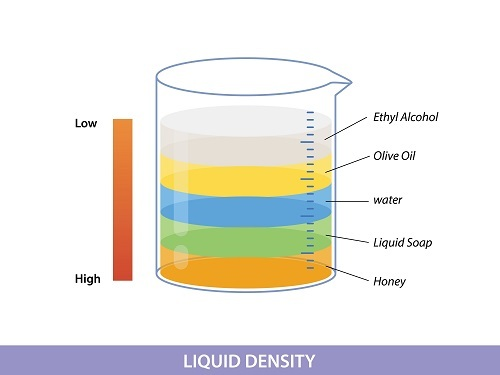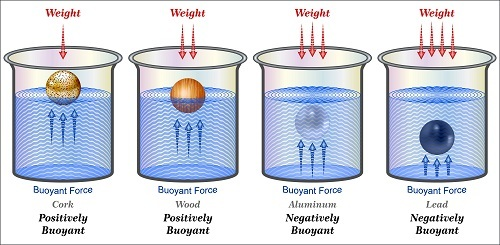# Density of Common Substances

## Introduction

Density of common substances is an important scientific term with many applications in human life and has a close association with mass. Every matter or the substances that surround us have a mass and that details the quantity or the amount of matter present in that particular substance. Density also has the name specific mass and volumetric mass. Density details the mass and volume of substances taken.

The precise measurements of density or the knowledge about density have been very limited during the past centuries. The invention made by Archimedes is responsible for the current facts about density. It is represented with the help of the Greek letter ρ and also commonly represented with the Latin letter D.

## What is Density?

For different substances with the same volume, it is found to have heaviness is different for example, the heaviness of water is more than that of wood. The quantities of mass and volume are two extensive properties, and the division of any two extensive properties are intensive properties. So, the ratio of mass and volume is an intensive property and is called density. Density is defined as the amount of anything in a unit volume for a particular substance. As it greatly depends on the mass other than the volume, it is given with the name mass density too.## Density-Formula

Density is the ratio of mass and volume and is represented by the Greek letter ρ, (rho), and is also a proportionality constant that details the density of any substance. The formula is,

$$\mathrm{\rho=m/V}$$

Where

m=mass

V=volume

The respective units of these quantities are, mL or cm^3 for volume and gram for mass. So, the unit of the intensive property, density, is g /mL or g/cm3.

## Density-Examples

The matter that surrounds us is very different in many ways. There are a wide variety of matters with a wide range of densities. The range of densities occupied by different matter range from a large value to even zero. The larger range of density values represents the number of substances that can be packed in a particular volume. As the packing of molecules in a defined volume increases, the density also increases to a great extent.

Among all the matters with different states, the ones with high density are solids than liquids and the least were gases. The density of gases also depends on some external factors, such as temperature and pressure. It is also found to greatly depend on the molecular weight of corresponding atoms present in that atom too. The range of densities of liquids is in an intermediate range. The density of liquids doesn't depend on the external factor of pressure, but they are sensitive to temperature. Solids are found to be packed heavily and have greater density than all the states of matter. Some solids that contain voids are also less dense, such as polyurethane foam has less density.

A list of densities of some common substances is tabulated in the below table.

SubstanceDensity (g/ mL)
Hydrogen0.09
Dry air1.29
Carbon dioxide1.98
Chlorine3.21
Olive oil0.92
Water0.997
Sulphuric acid1.83
Mercury13.55
Lithium0.53
Ice0.920
Sugar1.59
Sodium chloride2.16
Aluminium2.7
Iron7.9
Gold19.3
Osmium22.6

Table-1: List of substances and their corresponding density

## What is Relative Density

Relative density also given by the name specific gravity compares the density of a particular substance with a reference material. The reference substance used is commonly water. The equation used for calculating relative density (RD) is,

$$\mathrm{RD=\rho_{substance}/ \rho_{reference}}$$

The value of relative density can be obtained by taking the ratio of the substance's density that has been determined previously with the value of density of the reference material.

• If the calculated value of relative density is less than one, then it will be less dense than the reference material.

• If the calculated value of relative density is greater than one, then the substance will be dense than the reference material.

• If the calculated value of relative density is one, then the densities are equal.

Relative density is also a dimensionless quantity, as the corresponding ratios of densities are taken. Dry air is often used for calculating relative density in the case of gaseous materials. The buoyancy of fluid substances can be determined with the help of relative density. In the industrial sector, relative densities are used for the determination of the concentration of substances.

## Archimedes Principle

The physical law of buoyancy is Archimedes' principle. The principle is associated with the forces that have been applied to a particular object that has some fluids surrounding it. The application of force on these types of materials is found to reduce the weight of that particular object, too. This principle that deals with force were discovered by Archimedes of Syracuse. This principle states that for anybody that is partially or completely immersed in a gas or liquid (fluid), that is in rest is acted upon by an external buoyant force, the magnitude of the force applied to it is equal to the weight of the fluid displaced by that material. So that the buoyant force will then match the weight of that particular body.## Conclusion

Density is a term associated with the mass and volume of substances. In our surrounding system, the range of densities of the matter is very wide. Density is calculated by dividing two extensive properties, mass and volume. And density is an intensive property.

For substances, the one with a higher density is solids than liquids and then only gases. But certain variations are also possible because of the presence of voids and incompatible packing in some solids. Relative density is another term associated with density and has many applications in the industrial sector. Archimedes demonstrated a physical law of buoyancy that is related to the density of fluids.

## FAQs

Q1. Which metal has less density?

Ans. Even though, solids have higher density compared to all the states of matter. Lithium metal is found to be less dense. The density of lithium is 0.53 g/mL only. This is because there is no compact packing in the case of lithium.

Q2. What is the effect of temperature on the densities of matter?

Ans. The application of heat or the rise in temperature results in the expansion of substances. The application of heat causes the same mass species to have a greater volume. This phenomenon is called thermal expansion. It is mostly seen in gases as it is highly temperature sensitive. While in solids, the rate of expansion is low.

Q3. What are lava lamps?

Ans. Lava lamps are an application of density. It is also a fluid motion lamp that illustrates the density of species. It consists of a container that contains water and an oily organic liquid. As these two components do not mix, the density of the organic liquid is high. The application of heat makes the oily liquid less dense and then rises to the top from the bottom. In this way, the density of species is illustrated.Q4. Give the density of seawater.

Ans. For seawater, the density is found to be 1027 kg/m3. The unit of mass is here taken as kilograms.

Q5. Why is there no absolute density for water?

Ans. Water is a liquid and is highly sensitive to temperature. The value of the density of water varies with changing temperatures. So, water has no absolute density. The value of density in the case of water is 1g/cm^3 and is at a temperature of 4°C.

Updated on: 28-Apr-2023

404 Views